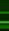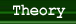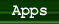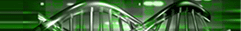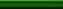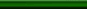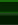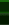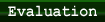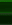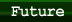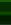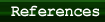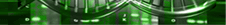### Theory

DNA computing has certainly generated a lot of excitement, but there is a reason that it has not yet replaced silicon as the primary platform for computing. nearly 50 years have been spent developing techniques and tools for programming traditional computers, and little of that effort can be easily transferred to DNA computing. Traditional computer programs run on processors specifically designed to run many different kinds of programs. DNA, however, has a very different purpose: guiding life. Since it is not yet possible to adapt the tools of DNA to our purposes (almost all useful biological molecules have been found in nature, rather than designed by humans), we must instead adapt our programs to the tools available. Since the computational capabilities of DNA are far more restricted than those of a modern silicon processor, we have to encode our problems and algorithms in very unnatural ways. It is not entirely unlike translating an algorithm to run on a minimal Turing machine.

It is interesting to compare DNA computing to the Turing Machine. Two things are needed to build a universal computer: storage and processing. In the case of the Turing Machine, the storage takes the form of the tape. In DNA computing, the DNA itself is a storage medium, one of the densest known. The processing of the Turing Machine is done by what Turing called the "Finite Control," or what we might think of as the state machine. The processing of DNA computing is a bit more complicated, and explained in the demo.

So if DNA computing takes so much effort, why is it such a hot topic? The immense power of DNA can be summarized with two words: massive parallelism. It is generally believed that so-called "NP" problems, like the Hamiltonian Path Problem, are fundamentally exponential. That is to say, no matter what algorithm is used, the amount of computation required to solve a given problem will be proportional related to an exponential function of the size of that problem. "An exponential amount of computation" is normally interpreted to mean that a given computer will take an exponential amount of time to solve, it. However, some algorithms can be "parallelized," which means that the computation is divided up between multiple independent computers. If a parallelizable algorithm is used, then instead solving the problem with an exponential amount of time on one computer, one can solve it using a fixed amount of time on an exponential number of computers. This is called parallel computing and is what gives DNA computing it's power. While an exponential amount of time on a conventional computer can add up fast, an exponential number of "DNA computers" really just means adding more DNA to the beaker. For large enough problems, the amount of DNA required can get unwieldy, the theory is that for some problems, properly encoded, it will be easier to get the amount of DNA required to solve the problem than the amount of computer time that would be required with traditional computers. It is interesting to note that, according to Adleman, the most tedious part of the process is the filtration by magnetic bead. In the Hamiltonian Path Problem, this step needs to be done once for every node, except the first and last. Therefore, while the heavy computation is done in constant time by an exponential amount of DNA, the extraction of the answer still takes a linear amount of time, not constant.

So when will DNA computers replace silicon? I think never. While DNA is capable of amazing feats of parallelism that have definite applications in supercomputing, the versatility, availability, durability, quick responsiveness, and ease of interface of silicon far outweighs the benefits of DNA computing in a desktop, server, or embedded environment. However the information density of DNA does make it an attractive medium for mass storage.